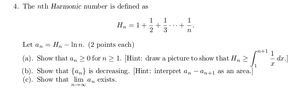# Calculus 3 (Series) UNSOLVED PROBLEM

• MHB
matdac
i have attached the problem set.

I have done the first three problems but number 4 is very difficult.

Can someone help me out?

Thanks

View attachment 7411

[Editor's note: The PDF below contains the complete problem set from which #4 is as shown above.]

#### Attachments

• Homework02.pdf
24.3 KB · Views: 13
•Question4.png
9.9 KB · Views: 24

Gold Member
MHB
Hello matdac and welcome to MHB! :D

We ask that our users show their progress (work thus far or thoughts on how to begin) when posting questions. This way our helpers can see where you are stuck or may be going astray and will be able to post the best help possible without potentially making a suggestion which you have already tried, which would waste your time and that of the helper.

Can you post what you have done so far?

Gold Member
MHB
i have attached the problem set.

I have done the first three problems but number 4 is very difficult.

Can someone help me out?

Thanks

[Editor's note: The PDF below contains the complete problem set from which #4 is as shown above.]

If you want to show that \displaystyle \begin{align*} H_n - \ln{ \left( n \right) } \geq 0 \end{align*} then you need to show that \displaystyle \begin{align*} H_n \geq \ln{ \left( n \right) } \end{align*}.

Can you at least evaluate \displaystyle \begin{align*} \int_1^{n+1}{ \frac{1}{x}\,\mathrm{d}x} \end{align*} and see WHY it might be important to draw the picture you have been told to?